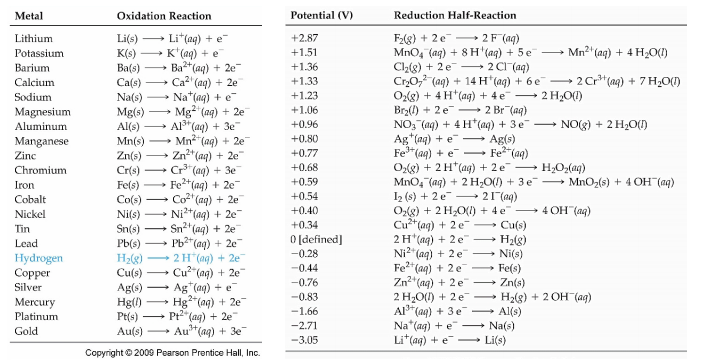# Problem: Using the table below, which substance can oxidize I – (aq) to I2 (s)?a) Br2 (l)b) Ni2+ (aq)c) Ag (s)d) Cu2+ (aq)e) More than one of the above

###### FREE Expert Solution
82% (363 ratings)
###### Problem Details

Using the table below, which substance can oxidize I – (aq) to I2 (s)?

a) Br2 (l)

b) Ni2+ (aq)

c) Ag (s)

d) Cu2+ (aq)

e) More than one of the aboveWhat scientific concept do you need to know in order to solve this problem?

Our tutors have indicated that to solve this problem you will need to apply the Redox Reactions concept. You can view video lessons to learn Redox Reactions. Or if you need more Redox Reactions practice, you can also practice Redox Reactions practice problems.

What is the difficulty of this problem?

Our tutors rated the difficulty ofUsing the table below, which substance can oxidize I – (aq) ...as low difficulty.

How long does this problem take to solve?

Our expert Chemistry tutor, Jules took 2 minutes and 51 seconds to solve this problem. You can follow their steps in the video explanation above.

What professor is this problem relevant for?

Based on our data, we think this problem is relevant for Professor Dixon's class at UCF.